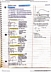# PHYSICS 1D03 Lecture Notes - Angular Momentum, Hockey Puck, Kinetic Energy

76 views5 pagescolin.menzies and 158 others unlocked53
Verified Note
53 documents

## Document Summary

Chapter 11, problems 11, 13, 15, 19, 55. Two astronauts are held together by a long rope and rotate about their common center of mass. One astronaut gathers in 1/3 of the rope separating them. Which of the following remains constant: kinetic energy, angular velocity, angular momentum, tension in the rope. Angular momentum of a particle rprl m = = ( v. This is the fundamental definition of l: l is a vector. z. O: like torque, it depends on the choice of origin (or pivot ). x, if the particle motion is all in the x-y plane, l is parallel to the z axis. y r v m. = mvr sin , etc v r v m. For a particle travelling in a circle (constant |r|), v = r , and. A hockey puck slides in a straight line at constant speed past a physicist at o. A) increases, then decreases: decreases, then increases.

## Get access

\$8 USD/m\$10 USD/m
Billed \$96 USD annuallyHomework Help
Study Guides
Textbook Solutions
Class Notes
Textbook Notes
Booster Class
Class+
\$8 USD/m
Billed \$96 USD annuallyHomework Help
Study Guides
Textbook Solutions
Class Notes
Textbook Notes
Booster Class